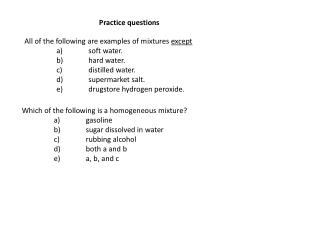DownloadDownload PresentationAll of the following are examples of mixtures except a ) soft water. b ) hard water.

# All of the following are examples of mixtures except a ) soft water. b ) hard water.

Télécharger la présentation## All of the following are examples of mixtures except a ) soft water. b ) hard water.

- - - - - - - - - - - - - - - - - - - - - - - - - - - E N D - - - - - - - - - - - - - - - - - - - - - - - - - - -
##### Presentation Transcript

1. Practice questions All of the following are examples of mixtures except a) soft water. b) hard water. c) distilled water. d) supermarket salt. e) drugstore hydrogen peroxide. Which of the following is a homogeneous mixture? a) gasoline b) sugar dissolved in water c) rubbing alcohol d) both a and b e) a, b, and c

2. Which one of the following lists gives the correct symbols for the elements • phosphorus, potassium, silver, chlorine, and sulfur? a) K, Ag, Po, Cl, S b) P, Po, Ag, Cl, S c) Ph, K, Ag, S, Cl d) P, K, Ag, Cl, S e) Ph, Po, Ag, Cl, S How many protons are there in the chromium-52 nuclide? a) 23 b) 24 c) 28 d) 29 e) 76 There are three isotopes of carbon differing with respect to a) neutrons. b) atomic number. c) nuclear charge. d) electron configuration. e) number of protons.

3. The average atomic mass of Eu is 151.96 amu. There are only two naturally occurring isotopes of europium, 151Eu with a mass of 151.0 amu and 153Eu with a mass of 153.0 amu. The natural abundance of the 131Eu isotope must be approximately a) 20%. b) 40%. c) 50%. d) 60%. e) 80%. The elements in a row of the periodic table are known as a) a family. b) a group. c) metals. d) metalloids. e) a period.

4. Ammonia can be made by reaction of water with magnesium nitride: __ Mg3N2(s) + __ H2O(l) → __ Mg(OH)2(s) + __ NH3(g) When the equation is properly balanced, the sum of the coefficients is a) 6. b) 8 c) 9. d) 12. e) 14. What is the formula mass of calcium phosphate, Ca3(PO4)2? a) 135.05 g/mol b) 215.21 g/mol c) 310.18 g/mol d) 279.20 g/mol e) 430.41 g/mol

5. How many atoms are present in 497 g of KPF6 (MM = 184.1 g/mol)? a) 2.70 × 1021 b) 1.63 × 1021 c) 2.39 × 1025 d) 1.22 × 1025 e) 1.30 × 1026 An organic compound has a molecular mass of 334.7 amu and contains 64.54% carbon by mass. How many carbon atoms are in each molecule of this compound? a) 17 b) 18 c) 22 d) 24 e) 28

6. Maleic acid contains 41.4% carbon, 3.47% hydrogen, and 55.1% oxygen by mass. A 0.050-mol sample of this compound weighs 5.80 g. What is the molecular formula of maleic acid? a) CHO b) C2H2O2 c) C3H3O d) C4H4O4 e) C8H8O8

7. Review calculations for the lab of determination of a mixture, and the relevant OWL problems Review the chemical analysis problems, limiting reagents and percent yield calculations, given reactants